# Entanglement of chaotic systems

### Background: thermalisation in quantum mechanics

Time evolution in quantum mechanics is unitary and therefore completely deterministic. Naively, one would, therefore, expect that quantum systems never thermalise. However, in experiments we only have access to a very small number of degrees of freedom of a quantum system and the question is whether that small subset sees thermalisation.

Two scenarios are possible:

1. Thermalisation occurs: eigenstate thermalisation hypothesis (ETH) holds, eigenstates tend to thermal states at long times, observables tend to their prediction from microcanonical ensemble. Information about initial conditions is long at long times. Entanglement entropy has extensive (volume law) behaviour.
2. Thermalisation does not occur: ETH does not hold, information is held indefinitely within locally accessible observables. Entanglement entropy behaves sub-extensively (area law).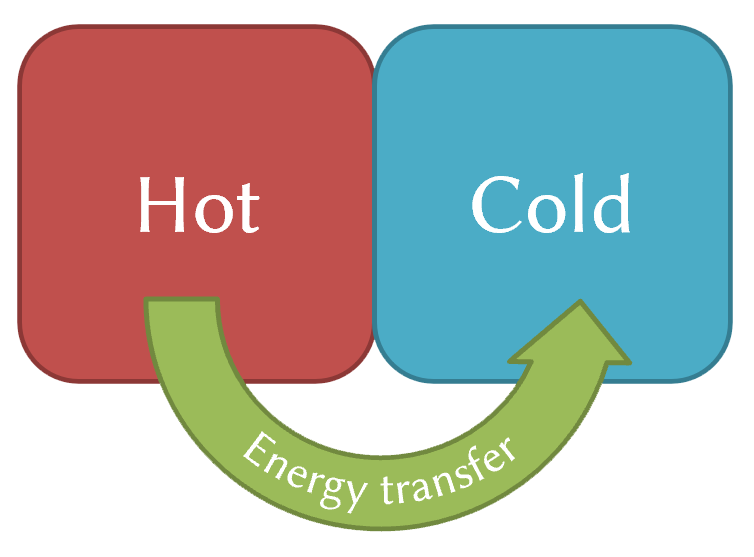Figure 1. A diagram of thermalisation in classical physics.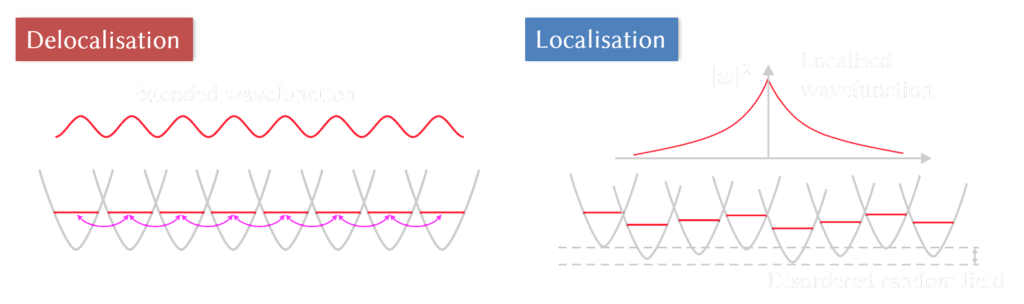Figure 2. Two scenarios: thermalisation occurs and the information is washed out throughout the whole system, or thermalisation does not occur and the wavefunction is localised (picture adapted from A. Aspect, M. Inguscio, Physics Today 62, 8, 30 2009).

### Weak measurements can cause entanglement transition

It was recently shown that measurements can be used to cause a quantum Zeno effect: frequent application of measurements can cause localisation in the system. One signature of that is the change from the volume law entanglement behaviour to the area law. In our work, we wanted to see how this situation changes if one uses weak (generalised) measurements: measurements where we have control over how much the system is disturbed by the measuring apparatus (see Fig. 3 for an example implementation).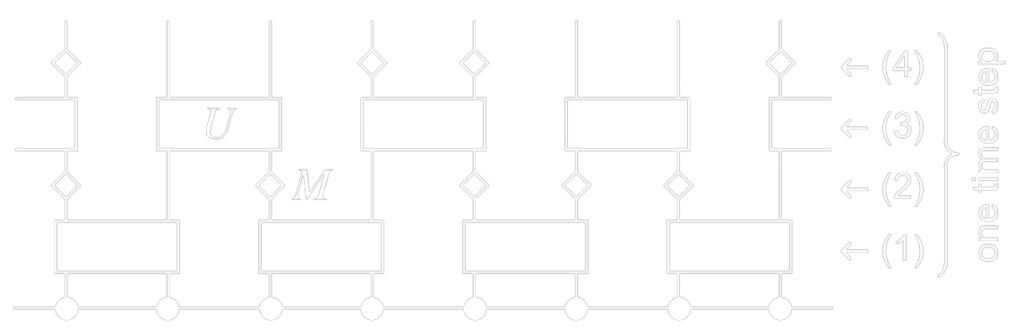Figure 4. Quantum circuit. Wavefunction is subjected to random unitary evolution $U$ and weak measurements $M$.

The model consists of a quantum circuit (see Fig. 4), where an initial wavefunction is subjected to the following operations:

• Unitary evolution $U$, which is implemented as random matrices from the Haar measure. This process entangles nearby spins and will favour the volume law.
• Weak measurements $M$ of a certain strength $\lambda/\Delta$ and applied with frequency $p$. Measurements, if strong enough, will break the entanglement and will favour the area law.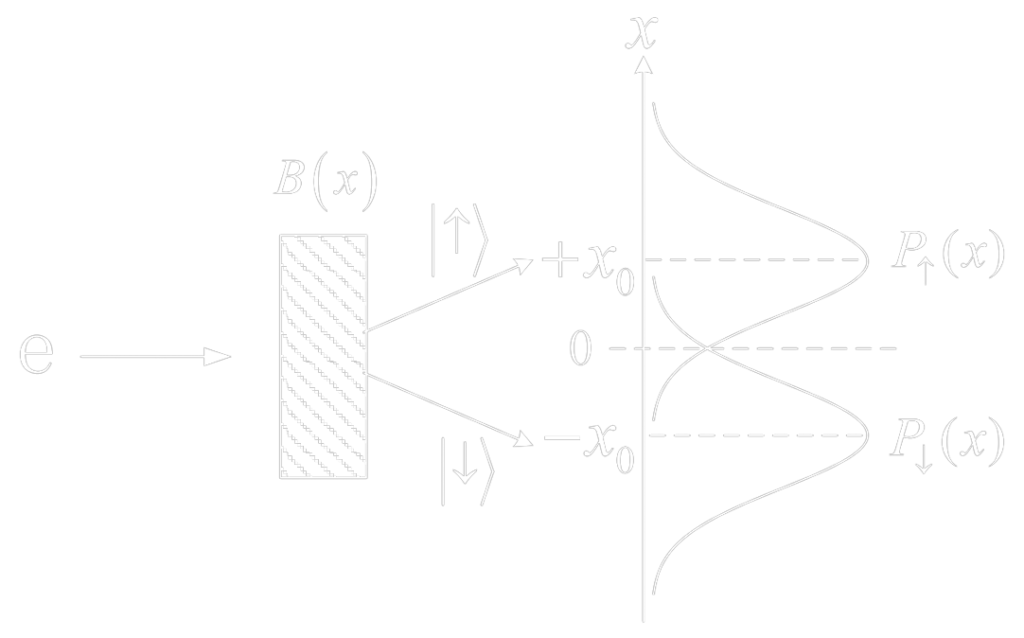Figure 3. Example implementation of weak measurements: Stern-Gerlach experiment with weak interactions between electrons and the magnetic field causes smearing on the screen.

By investigating multiple quantities, namely entanglement entropy $S$, fluctuations of entropy $\text{var}(S)$, and mutual information $I(A:B)$, we have shown that there is a critical value of measurement strength below which there is always a volume law irrespective what the measurement frequency is. There is a similar critical value for the frequency as well.

We have also estimated where the transition line lies as a function of measurement strength $\lambda/\Delta$ and measurement frequency, $p$ as shown in Fig. 5.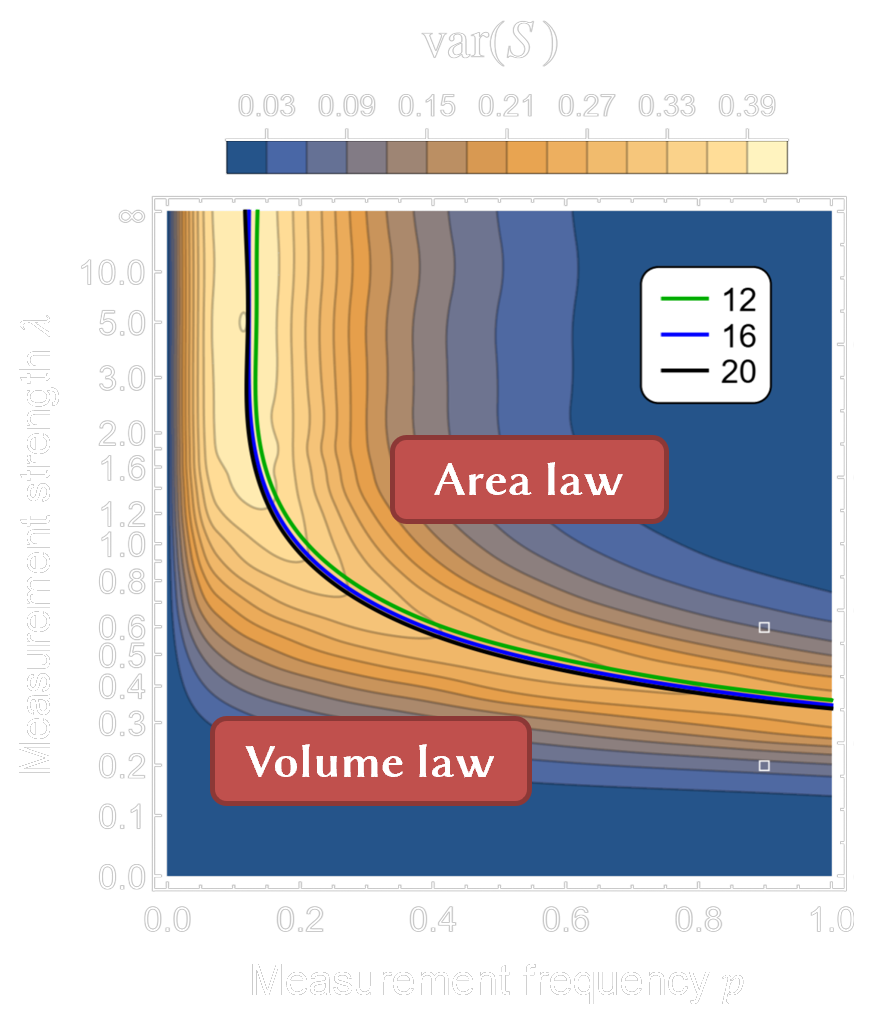Figure 5. Fluctuations of the entanglement entropy $var(S)$ reveal the transition line between the area law and the volume law.

### Many-body localisation of zero modes

We wanted to observe if zero modes exhibit interesting properties in the many-body localised phase. To do this, we selected a spin-1 system with chiral symmetry, with the following Hamiltonian:

$$H = \sum_{i=1}^N S^x_i S^x_{i+1} + \sum_{i=1}^N h_i S^z_i,$$

where $S^n$ are the spin-1 operators, and the on-site potentials are disordered $h_i\in[-W,W]$.

We first determined the transition point between the ergodic and localised regimes, utilising the ratio of the nearby level spacings $\langle r\rangle$ as shown in Fig. 6.

Then, we compared the entanglement entropy histograms between zero modes and non-zero modes in the localised phase (see Fig. 7). This reveals an interesting difference, namely, there are different peaks in both histograms, which correspond to different dimers. Therefore we concluded that many-body localisation causes the production of different dimers between the zero and non-zero modes.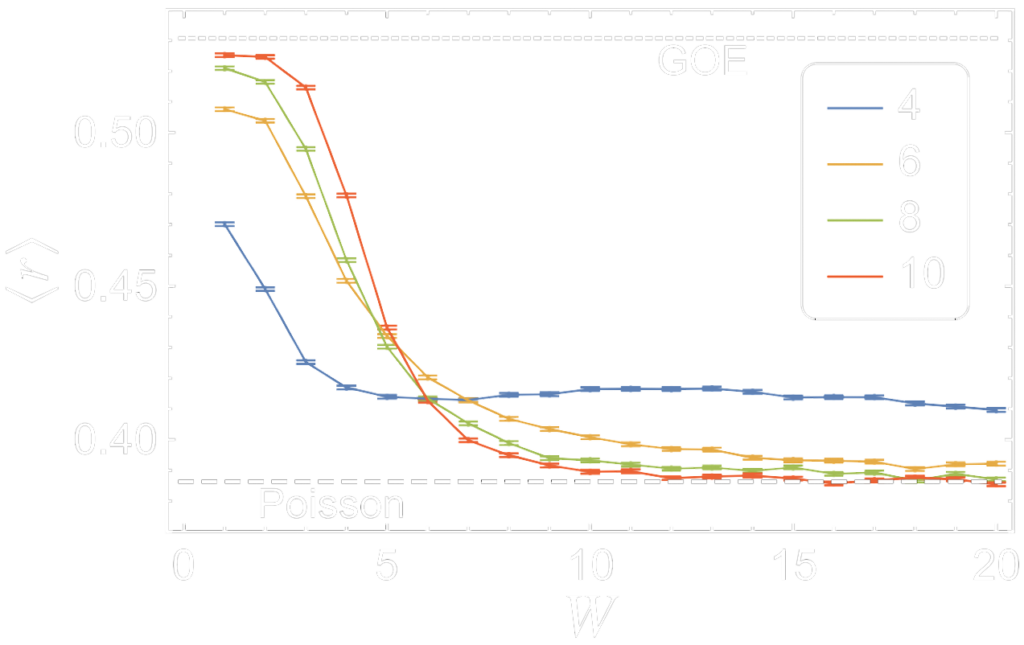Figure 6. Ratio of the nearby level spacings $\langle r\rangle$ can be used to estimate the transition region.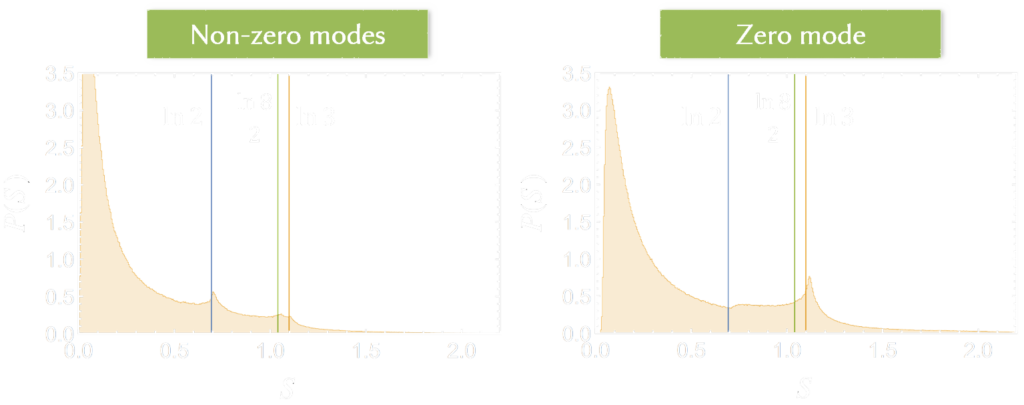Figure 7. Comparison between the entanglement entropy histogram for non-zero and zero modes. Dimers corresponding to the $\ln3$ are suppressed for the non-zero modes, while in the zero modes there are no $\ln2$ dimers.

### The universality of the entanglement transition – linking stroboscopic to continuous measurements

Although the transition from the volume law to the area law phase has been thoroughly investigated for the stroboscopic circuit, the corresponding transition for the continuous case was still a matter of debate. In order to address this, we employed two tricks to get to the continuous-time limit:

• We use weak measurements with the measurement strength scaled as $\lambda = \lambda_0 \sqrt{dt}$.
• Instead of completely random unitary matrices from the Haar measure, we use unitary matrices generated by the Poisson kernel with an internal parameter $\mu \sim \sqrt{dt}$. These matrices will range from fully random $(\mu=1)$ to an identity $(\mu=0)$.

When $\mu\to\infty$, we reach the continuous-time limit. Interestingly, one can show that the stroboscopic and the continuous regimes are smoothly connected (see Fig. 8), which suggests that both phase transitions are of the same nature.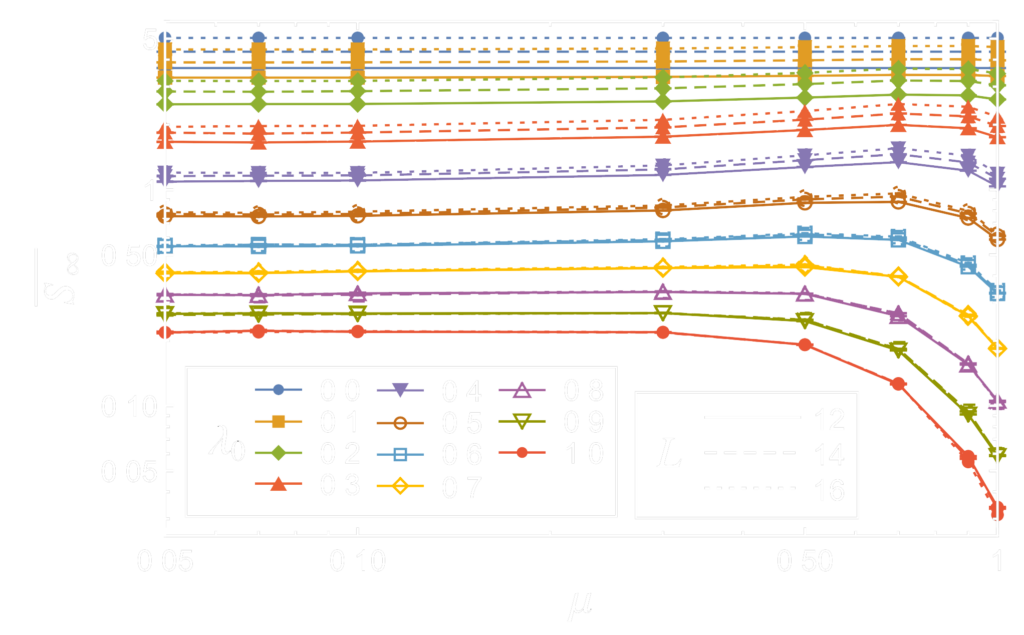Figure 8. Averaged entanglement entropy $\overline{S}_\infty$ as a function of time step variable $\mu$. The plot shows a smooth connection between the stroboscopic $\mu=1$ and continuous $\mu\to0$ case.